# Short Notes: Dielectrics and Capacitors# Dielectrics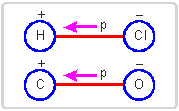The substances containing no free charges are celled dielectrics. Each dielectric possesses positive and negative charges both, but they are not free and their algebraic sum is zero. [i.e.  Σq = 0 for a dielectric and any charge q is not free]. The dielectrics is of two types

(i) Polar dielectrics: In some dielectrics the centres of positive and negative charges do not coincide, so that dielectric has permanent electric dipole moment, such dielectrics are called polar dielectrics. The examples are H2o, HCl, CO, etc.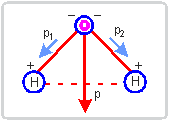(ii) Non polar dielectrics: In some other dielectrics the centres of positive and negative charges coincide, so that the dielectric does not possess a permanent electric dipole moment; such dielectrics are called non polar. The examples are carton dioxide CO2, hydrogen H2, etc.

# Electric Polarisation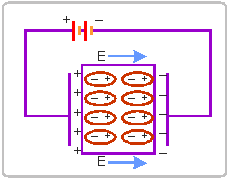When a dielectric is placed in an external electric field, the centres of positive and negative charges get separated (in non polar dielectrics) and get farther away (in polar dielectrics), so that the molecules of dielectric gain a electric dipole moment. This process is called polarisation and the dielectric is said to be polarised.

The induced dipole moment per unit volume is called polarisation i.e.

ρ = σP

The induced dipole moment is usually proportional to electric field applied, i.e.,.

Where α is called molecular (or atomic) polarisability. The unit of polarisability . α is m3

If is the number of molecules per unit volume, the polarisation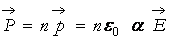## Capacitors and Capacitance

A capacitor contains two oppositely charged metallic conductors at a finite separation. It is a device by which capacity of storing charge may be varied simply by changing separation and/or medium between the conductors.

The capacitance of a capacitor is defined as the ratio of magnitude of charge (Q) on either plate and potential difference (V) across the plate, i.e.,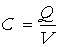The unit of capacitance is coulomb/volt or farad (F).

## Combination of Capacitors in Series and Parallel

If the capacitor of required capacitance is not available, then two or more capacitors may be combined to provide the required capacitance. There are two main methods of combination:

1. Series Combination: To reduce the capacitance, the capacitors are connected in series. In this combination, the first plate of first capacitor is connected to electric source, the second plate of first capacitor is connected to first plate of second capacitor, the second plate of second capacitor is connected to first plate of third capacitor and so on; the second plate of last capacitor is connected to earth. In fig. (a) three capacitors of capacitances  C1,C2,C3 are connected in series between points A and D.Suppose by means of electric source a charge +Q is given to the first plate of first capacitor C1. By induction ?Q charge is induced on the inner surface of second plate of first capacitor and a charge +Q on inner surface of first plate of second capacitor C2 and so on (fig. a). Thus first plate of each capacitor has charge +Q and second plate of each capacitor has charge ?Q.

Let the potential differences across the capacitors C1,C2,C3  be V1,V2,V3  respectively. As the second plate of first capacitor C1 and first plate of second capacitor C2 are connected together, their potentials are equal. Let this common potential be VB Similarly the common potential of second plate of C2 and first plate of C3 is VcThe second plate of capacitor C3 is connected to earth, therefore its potential C1 = 0 As charge flows from higher potential to lower potential, therefore  V> V> V> VD .Adding equations (1), (2) and (3), we get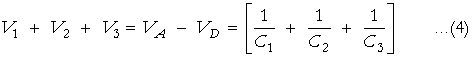If V be the potential difference between A and D, then

VA −  VD  = V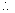From (4), we getIf in place of all the three capacitors, only one capacitor is placed between A and D such that on giving it charge Q, the potential difference between its plates become V, then it will be called equivalent capacitor. If its capacitance is C, thenComparing (5) and (6), we getThus in series arrangement, The reciprocal of equivalent capacitance is equal to the sum of the reciprocals of the individual capacitors.

2. Parallel Arrangement: To increase the capacitance, the capacitors are connected in parallel. In this combination the first plate of each capacitor is connected to a common point A and second plate to another common point B. The point A is connected to electric source and point B is connected to earth. In fig. (a) three capacitors of capacitance C1,C2,C3  are connected in parallel.Let a charge Q be given to point A by the means of an electric source. The first plate of each capacitor will be at potential A and second plate, at zero potential, because it is connected to earth. Clearly the potential difference between plates of each capacitor

VA −  VB = V= V (say)

The charge Q will be divided on capacitors C1,C2,C3 .

Let q1,q2,q3  be the charges on capacitors C1,C2,C3  respectively.

Then   Q = q1 + q2 + q3         …….(1)

q1 = C1V, q2 =  C2V , q3 =  C3V

Substituting these values in (1) , we get

Q = C1V + C2V + C3V

or

Q = (C1 + C2 + C3)V      ………(2)

If, in place of all the three capacitors, only one capacitor of capacitance C be connected between A and B; such that on giving it charge Q, the potential difference between its plates be V, then it will be called equivalent capacitor. If C be the capacitance of equivalent capacitor, then

Q = CV     …… (3)

Comparing equation (2) and (3) , we get

CV = (C1 + C2 + C3)V

or        C = (C1 + C2 + C3)                  …….(4)

# Capacitance of Parallel Plate Capacitor

A parallel plate capacitor consists of two parallel metallic plates separated by a dielectric. The capacitance of parallel plate capacitor is given by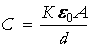where K is dielectric constant,area of each plate and = separation between the plates.

Special Cases: (i) When there is no medium between the plates, then  K =1 ,so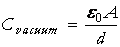(ii) When space between the plates is partly filled with a medium of thickness t and dielectric constant K, then capacitanceEffect of introducing a dielectric between plates of a charged parallel plate capacitor

 S.No. Physical Quantity When battery remains connected When battery is removed before introduction of dielectric (i) Capacitance (C) Increases K-times Increases K-times (ii) Charge (Q) Increases K-times Remains constant (iii) Electric potential (V) Remains constant Decreasestimes (iv) Electric field Remains constant Decreasestimes (v) Electrostatic Energy Stored Increases K-times Decreasestimes

# Energy Stored in a Parallel plate Capacitor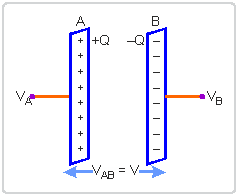When a capacitor is charged by a battery, work is done by the charging battery at the expense of its chemical energy. This work is stored in the capacitor in the form of electrostatic potential energy.

Consider a capacitor of capacitance C. Initial charge on capacitor is zero. Initial potential difference between capacitor plates = zero. Let a charge Q be given to it in small steps. When charge is given to capacitor, the potential difference between its plates increases. Let at any instant when charge on capacitor be q, the potential difference between its plates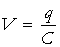. Now work done in giving an additional infinitesimal charge dqto capacitor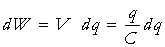The total work done in giving charge from 0 to Q will be equal to the sum of all such infinitesimal works, which may be obtained by integration. Therefore total workIf V is the final potential difference between capacitor plates, then  Q =CV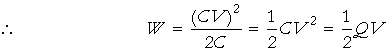This work is stored as electrostatic potential energy of capacitor i.e.,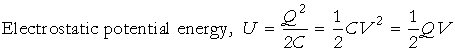# Energy Density

Consider a parallel plate capacitor consisting of plates, each of area A, separated by a distance d. If space between the plates is filled with a medium of dielectric constant K, thenIf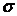is the surface charge density of plates, then electric field strength between the plates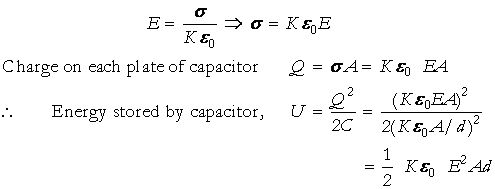But  Ad = τ, volume of space between capacitor platesThis is expression for electrostatic energy density in medium of dielectric constant K.

In air or free space  (K = 1)therefore energy density,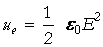.

# Van de Graaff Generator

It is a device used to generate high potential of the order of 10 million volts.

Principle: It is based on the following two electrostatic phenomena:

(i) The charge always resides on the outer surface of a hollow conductor.

(ii) The electric discharge in air takes place readily at the pointed ends of the conductor.